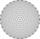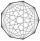# Planimetrics - math word problems

Study plane measurements, including angles, distances, and areas. In other words - measurement and calculation of shapes in the plane. Perimeter and area of plane shapes.

#### Number of problems found: 2231

• Triangle SAAThe triangle has one side long 71 m and its two internal angles is 60°. Calculate the perimeter and area of the triangle.
• TrapezoidCalculate the area of trapezoid ABCD with sides |AB|= 50 cm, |BC|=25 cm, |CD|=10 cm, |AD|=25 cm..
• Bicycle wheelA bicycle wheel has a diameter of 60 cm. Approximately how many times does the wheel rotate at 2.5 km long trip?
• N-gon anglesWhat is the sum of interior angles 8-gon? What is the internal angle of a regular convex 8-polygon?
• Triangle SSSCalculate perimeter and area of ​​a triangle ABC, if a=53, b=46 and c=40.
• Slope of trackCalculate the average slope (in permille and even in degrees) of the rail tracks between Prievidza (309 m AMSL) and Nitrianske Pravno (354 m AMSL), if the track is 11 km long.
• N-gonHow many diagonals have convex 11-gon?
• Right triangle AlefThe obvod of a right triangle is 84 cm, and the hypotenuse is 37 cm long. Determine the lengths of the legs.
• Rotation of the EarthCalculate the circumferential speed of the Earth's surface at a latitude of 61°​​. Consider a globe with a radius of 6378 km.A passenger car and an ambulance come to the rectangular crossroad, the ambulance left. Passenger car at speed 39 km/h and ambulance at 41 km/h. Calculate such a relative speed of the ambulance move to the car.
• Right triangleCalculate the missing side b and interior angles, perimeter, and area of ​​a right triangle if a=10 cm and hypotenuse c = 16 cm.

We apologize, but in this category are not a lot of examples.
Do you have an exciting math question or word problem that you can't solve? Ask a question or post a math problem, and we can try to solve it.

We will send a solution to your e-mail address. Solved examples are also published here. Please enter the e-mail correctly and check whether you don't have a full mailbox.